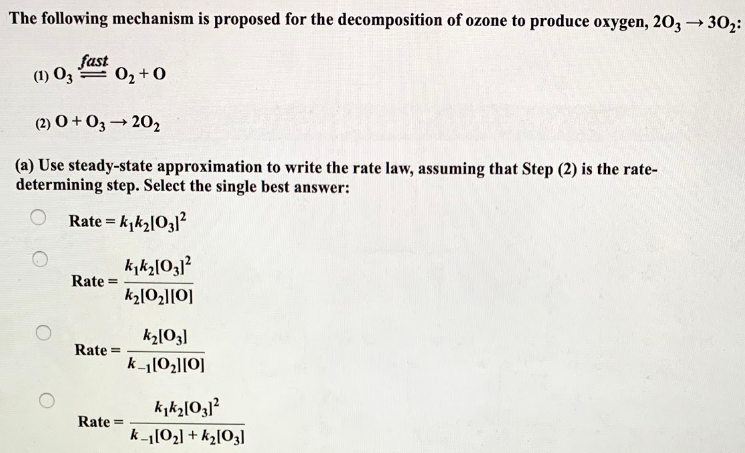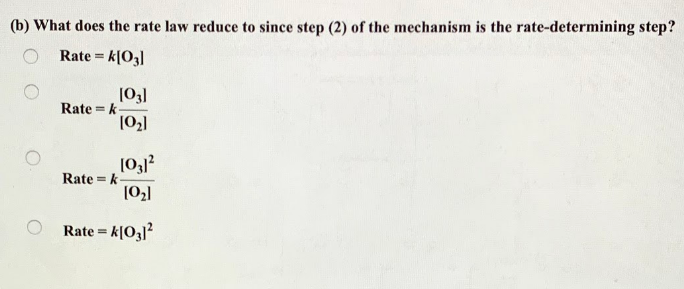# The following mechanism is proposed for the decomposition of ozone to produce oxygen, 2O3 → 3O2: (1) O3 ⇌ O2 + O (2) O + O3 → 2O2 (a) Used steady-state approximation to write the rate law, assuming that Step (2) is the rate-determining step. Select the single best answer: (b) What does the rate law reduce to since step (2) of the mechanism is the rate-determining step?